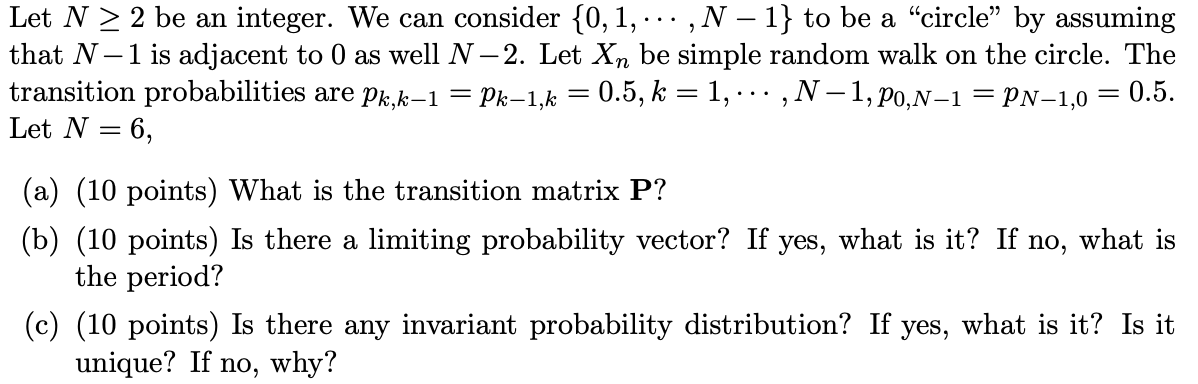Home / Expert Answers / Other Math / let-n2-be-an-integer-we-can-consider-0-1-n1-to-be-a-34-circle-34-by-assuming-that-n1-is-pa372

# (Solved): Let N2 be an integer. We can consider {0,1,,N1} to be a "circle" by assuming that N1 is ...Let be an integer. We can consider to be a "circle" by assuming that is adjacent to 0 as well . Let be simple random walk on the circle. The transition probabilities are . Let , (a) (10 points) What is the transition matrix ? (b) (10 points) Is there a limiting probability vector? If yes, what is it? If no, what is the period? (c) (10 points) Is there any invariant probability distribution? If yes, what is it? Is it unique? If no, why?

We have an Answer from Expert